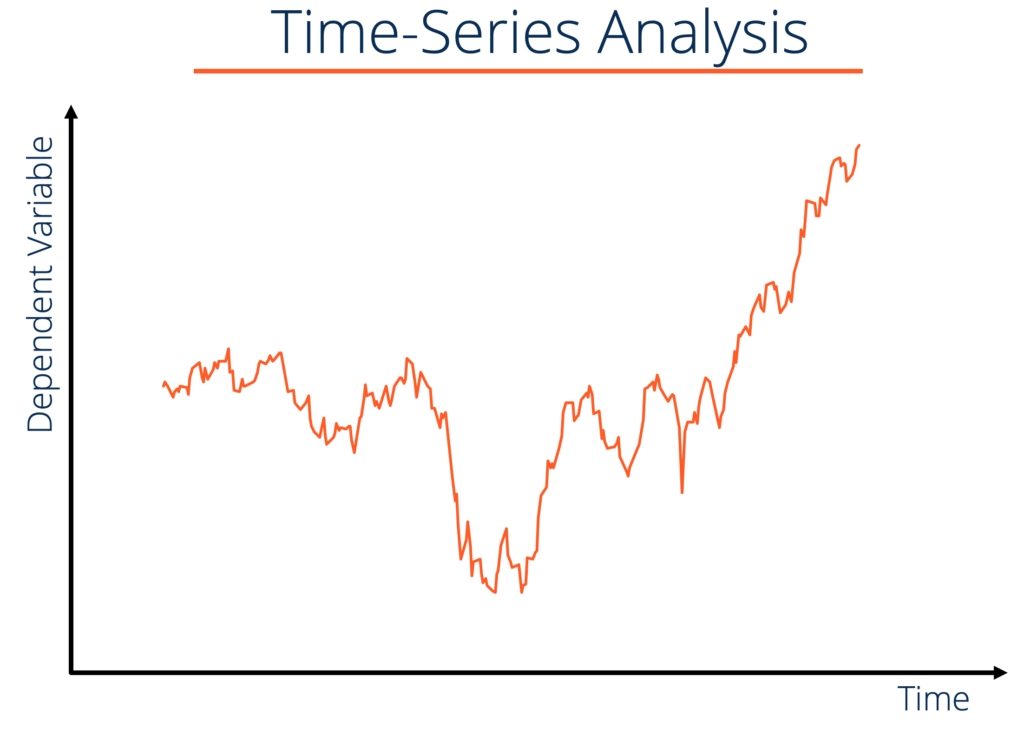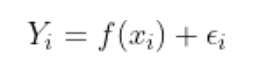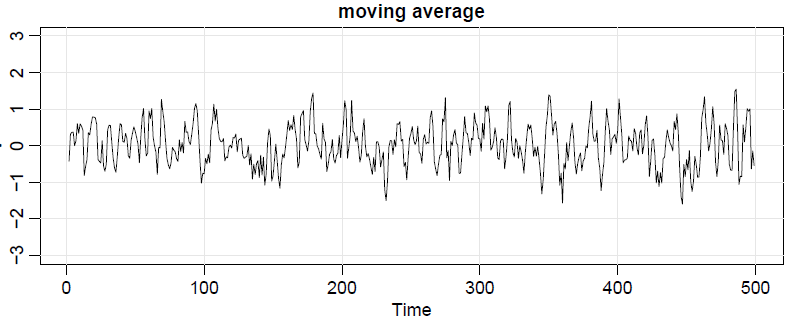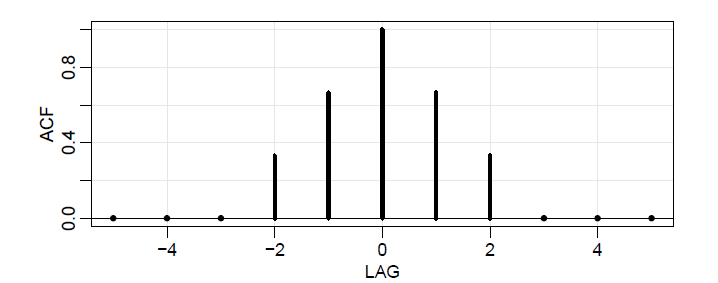# We discuss the following

• What is Time Series?
• What is the main focus of Time Series?
• How is Time Series different from Regression?
• How to mathematically model Time Series?
• Why stationarity of the Time Series?
• What is the Central Idea?
• What is ARIMA modeling in short?

# What is Time Series?

## Time series data is a sequence of data over discrete time points.are the data points over time, where 1,2,3,..,n are the time points.

In terms of probability, X is called a random variable.The data are collected at discrete time points, which are very close.
`Time series is an application of the Stochastic Process in probability.`

# What is the main aim of Time Series Analysis?

• Forecasting is the main focus of time series analysis by measuring and estimating the dependence among the data points.

This leads us to the next question.

# How is Time Series different from usual Regression?

## Regression FrameworkThere are n such data points. The aim is to approximate such a function f from the data.

One important assumption is that the errors are independent of one another, which in turn means that the data points are independent (white noise).

In time series, the errors are in fact dependent upon one another, as time proceeds, which is a really genuine assumption.

Thus, we cannot apply the usual regression techniques in Time Series Analysis.

# How to mathematically model Time Series?

## 2. Trend, Seasonality, and then Detrending of Time Series

The trend is actually the f(x), which captures the general overall behavior.

There are mathematical methods to identify the trend.

The relationship of the trend can be super complex why?

Because, one time series can be dependent upon other time series, with some extra component of its own trend, added with the relationship with other time series.

Yeah, it is complex in nature.

How to handle this?

The way to handle this is that we have to detrend (remove the trend) to get residuals and analyze it.

The most important aspect of the time series is not to model the trend, but to model the heavily dependent errors.

I will discuss one way of doing it.

• Differencing

We now try to remove the trend by subtracting the trend equation, and by difference.

Look into the pictures below.

Observe still there is some order in the detrended (by linear equation) while the first difference looks more random like an error.

This is because, by difference, we have also accounted for the seasonality aspect of the time series.

This forms the I aspect of the ARIMA model.

I will discuss this in detail later on in a different post.

Now, after detrending the whole time series, we are left with the residuals/sample errors, which have a dependence structure and they must have some good properties, which we will explore.

## 3. Dependence Structure (of errors of data points unlike regression)

The lack of independence between errors of two data points can be assessed numerically, as in classical statistics, using the notions of covariance and correlation.

## Autocovariance Function

The autocovariance function measures the dependence between errors of two data points at time t and time s.

But, covariance can be very large in magnitude. So, we need to normalize it, to make it autocorrelation function.

## Autocorrelation Function (ACF)

The autocorrelation function (ACF) measures the normalized dependence between two data points at time t and time s. It lies between 1 and -1. (Why?)

Understand, that we need to estimate the autocorrelation function from data using the sample correlation.

## - Good(Predictable) errors of Time Series is stationary

A time series (of errors) are behaving in a nice way if any collection of them are invariant over time (stationary) in a probabilistic sense.

These two sets of data points must behave similarly (stationary) in a probabilistic framework.

But, this hardly happens in real life and it is very hard to check.

So, we will focus on two conditions, where we can understand the first-order moment (mean) and the second-order dependence moment (covariance) behaviour.

• The mean value function must remain constant over time. We can subtract a constant from it to make it 0 always.
• The autocovariance function (the dependence structure) of two data points at time t and s, must be only dependent on the time lag |t-s|.
`Trend is not stationary, since the mean function is changing with time. So, we need to detrend to make it stationary.`

This implies that the dependence function of the errors is based on a good behavioral notion of stationarity.

We will learn various interesting methods to model this dependence using ACF.

In the case of stationarity, the ACF function reduces to a function of the time lag (h) only.

Why? Watch the video. :)

## - Autocorrelation Function (ACF) decreases over time.

This intuitively means, far apart data points are hardly correlated and dependent upon one another.

ExampleExample 1Lag = t-s, where we understand the dependence of data points, Xt and Xs by ACF. Observe that the estimated ACF function decreases with an increase in the magnitude of lag.

# Why stationarity of the Time Series?

The stationarity of time series helps us to estimate the dependence structure and the mean behavior of all the possible sample collection.

This is turn helps us to understand to how many degrees the data points are connected to each other (in terms of lag) and this forms the basis of the AR and MA aspect of the ARIMA model.

# What is the Central Idea?

## Step 1

`Transform data into stationary data.`
• In the case of polynomial trend, we can difference many times to detrend.
• In the case of exponential trend, we need to non-linearly transform the data into polynomial trend and then difference.
• There are other methods to detrend.
• There are other smoothing methods to understand the trend and then detrend (Nadarya Watson Estimator, etc).
• Check various plots to understand graphically and do some statistical checks to check stationarity.

We will learn these in detail in another post. Here, we essentially prepare the data for the understanding of the dependence structure of step 2.

## Step 2

`Model and Predict the dependence structure of the errors.`

The modeling of the dependence is done by the famous models like

• AR
• MA

# What is the ARIMA model in short?

• Change data into stationary data by transformation and differencing. (I)
• Apply AR and MA models to estimate the dependence structure of the differenced time series and predict.
• That’s AR + I + MA = ARIMA for you.